www.batmath.it

### Square matrices, determinants and inverses

#### Square matrices

A square matrix is a n×n matrix. The common number n of rows and columns is also called the order of the matrix. In the set of all matrices of order n, matrix product is an internal operation and the matrix whose entries are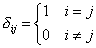is called the identity matrix, In, as it satisfies the following properties: for every given matrix A, A·In=In·A=A. In other words In is the neutral element for matrix product.

In this space it is also possible to define the k-th power of a matrix: A0=In, An=A·An-1.

A square matrix A of order n, is called non-singular or invertible if there exists a matrix B of order n such that

A·B = B·A =In.

The matrix B, if it exists, is unique and is called the inverse of A and is denoted by A-1.

#### Example

Find the inverse, if it exists, of the matrix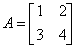. We must search a matrix of the form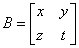such that the previous identity holds. This means we must solve the following linear system in four unknowns: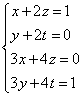. Via the Gauss-Jordan algorithm we easy find the solution: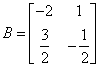.

The main properties of the inverse of a matrix are:

• (A-1)-1 = A

• (AB)-1 = B-1A-1

The problem of finding the inverse, if it exists, of a given matrix A, is very important. The technique we used in the previous example relates this problem to a question of solving a linear system. In some sense this is unsatisfactory, since it is not simple to solve such a system without a lot of work. So we now look for a different strategy.

First of all we try to generalize the previous example to a generic matrix of order two: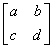.  We write down the system as in the example above and obtain the following augmented matrix: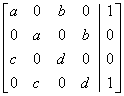.

• If a=c=0, the system has no solution.

• If a0, via the Gauss-Jordan algorithm we obtain the following reduced row-echelon form of the augmented matrix: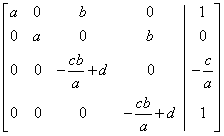. The system has one solution if (and only if) ad-bc0, and the solution gives the inverse matrix: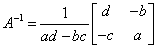.

• If c0 and a=0 we can interchange rows one and two with rows three and four and obtain the same result.

So the number ad-bc plays a special role in the theory of matrices of order two: if it is not zero the matrix has an inverse, otherwise it has no inverse.

#### Determinants

Given a generic matrix of order two,, the number ad-bc is the determinant of the matrix, det(A). The notation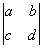is also used. If and only if det(A) is different from zero the matrix has a unique inverse. If we attempt to repeat the calculations above to matrices of order three, then it is very likely that we shall end up in a mess with possibly no firm conclusion. (Try it if you must!). We shall follow a different approach: precisely an inductive one. In other words we shall define the determinant of a matrix of order n in terms of determinants of matrices of order n-1: as we know the determinant of a matrix of order two this will lead us to the desired result.

A new definition is needed in order to simplify the notations.

Given a matrix A of order n and an entry aij we consider the new matrix, Aij, of order n-1 that we obtain if we delete the i-th row and j-th  column of A: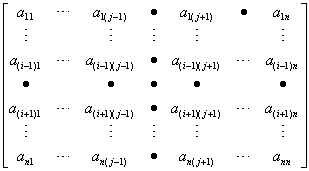. The number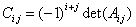is called the cofactor of the entry aij in the matrix A.

Given a row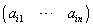or a column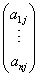one can prove (but it is not so easy!) that the following expressions are equal and independent of the row or column chosen: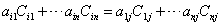. These expression are called cofactor expansions by row i or by column j. The common value of these expressions is called the determinant of the matrix A, denoted by det(A).

It is easy to check that this agrees with our earlier definition of the determinant of a matrix of order two.

#### Example

Find the determinant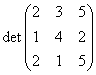. Let us use the cofactor expansion by row 1. Then: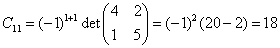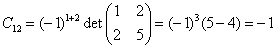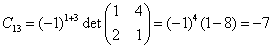so that det(A) = 2·18+3·(-1)+5·(-7) = -2.

#### Calculating the inverse of a matrix

In order to outline a procedure for finding the inverse of a matrix we need two more definitions.

Given a matrix A we call transpose of A the matrix the matrix, At, obtained by interchanging the rows and columns of the matrix A. For example if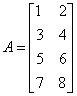, then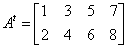.  If X is a one column matrix (a column vector),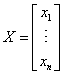, we have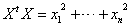, a property that will be useful in the following.

Given a matrix A the adjoint of A is the matrix, adj(A) is the transpose of the matrix of the cofactors of  the entries of A.

Now it can be proved that a matrix A is invertible if and only if det(A) is different from zero and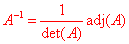.

#### Example

Given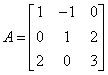, we have: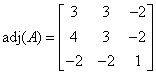. As det(A)=-1, it follows that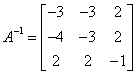.

first published on march 15 2002 - last updated on september 01 2003